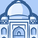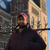QlikView App Dev

Discussion Board for collaboration related to QlikView App Development.

Announcements
QlikWorld 2022, LIVE in Denver CO., May 16-19, 2022. REGISTER NOW TO RECEIVE EARLY BIRD PRICING
cancel
Showing results for
Did you mean:Contributor III

AGGR Function Not working for Partial Sum Percentages

Good Morning,

I am developing an app in which the users requirements are to calculate the YoY % Growth for different sales categories by month. Since the user wanted to be able to expand and collapse field dimensions (i.e. Category and Month) I used a Pivot table to display the data. One nuance in the requirements was that the user wanted to be able to see Actuals and Forecast. (i.e. Actual sales would be represented by months that have already completed while current month and future months would be displayed as Forecast sales.) The SUM(AGGR( function works fine with the IF Statement I have in terms of 2018 sales, 2017 sales and YoY Sales Difference. However when I use the AGGR function for the YoY % Growth, the partial sum total is incorrect. In my example below the partial sum reads -40% when it should be +26%.

I need assistance getting correct value shown on the YoY % Growth line.

If anything is this request is confusing I'd be glad to help clarify.

-B-

1 Solution

Accepted SolutionsChampion

Hi,

try this as an expression for YoY %:

=(SUM(AGGR(IF(SUM({<YEAR={'2018'},SCENARIO={'ACTUALS'}>}UNITS)=0,SUM({<YEAR={'2018'},SCENARIO={'FORECAST'}>}UNITS),SUM({<YEAR={'2018'},SCENARIO={'ACTUALS'}>}UNITS)),MONTH, CATEGORY))

-

SUM(AGGR(IF(SUM({<YEAR={'2017'},SCENARIO={'ACTUALS'}>}UNITS)=0,SUM({<YEAR={'2017'},SCENARIO={'FORECAST'}>}UNITS),SUM({<YEAR={'2017'},SCENARIO={'ACTUALS'}>}UNITS)),MONTH, CATEGORY)))

/

SUM(AGGR(IF(SUM({<YEAR={'2017'},SCENARIO={'ACTUALS'}>}UNITS)=0,SUM({<YEAR={'2017'},SCENARIO={'FORECAST'}>}UNITS),SUM({<YEAR={'2017'},SCENARIO={'ACTUALS'}>}UNITS)),MONTH, CATEGORY))

3 RepliesChampion

Hi,

try this as an expression for YoY %:

=(SUM(AGGR(IF(SUM({<YEAR={'2018'},SCENARIO={'ACTUALS'}>}UNITS)=0,SUM({<YEAR={'2018'},SCENARIO={'FORECAST'}>}UNITS),SUM({<YEAR={'2018'},SCENARIO={'ACTUALS'}>}UNITS)),MONTH, CATEGORY))

-

SUM(AGGR(IF(SUM({<YEAR={'2017'},SCENARIO={'ACTUALS'}>}UNITS)=0,SUM({<YEAR={'2017'},SCENARIO={'FORECAST'}>}UNITS),SUM({<YEAR={'2017'},SCENARIO={'ACTUALS'}>}UNITS)),MONTH, CATEGORY)))

/

SUM(AGGR(IF(SUM({<YEAR={'2017'},SCENARIO={'ACTUALS'}>}UNITS)=0,SUM({<YEAR={'2017'},SCENARIO={'FORECAST'}>}UNITS),SUM({<YEAR={'2017'},SCENARIO={'ACTUALS'}>}UNITS)),MONTH, CATEGORY))Contributor III
Author

Youssef,

The formula worked perfectly. Thanks for your assistance!

-B-Champion

You're welcomeGood luckCommunity Browser